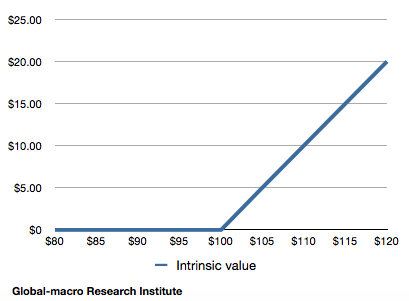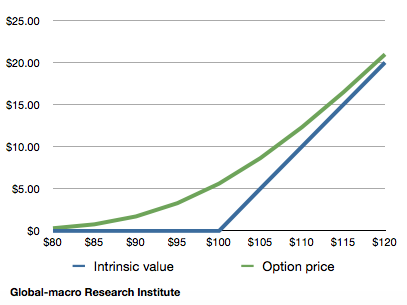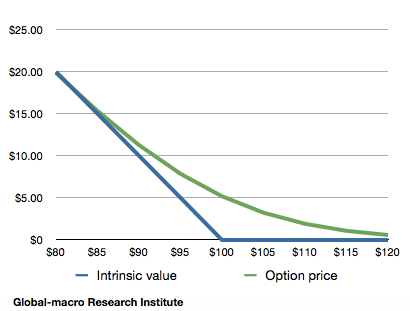# How to trade options : what are call options and put options?

This is the first article of a series, “How to trade options”, which explains how to trade options and what they are. First of all, we explain here the basics of call options and put options.

Options are a way to gain a profit in a market that neither constantly goes up nor goes down. With a long-lasting market trend such as stocks after 2008 or gold after 2012, bullish or bearish, you may simply buy or short sell a financial instrument.

On the other hand, when you know a market will not move for a while or when you know it will be volatile but do not know if it goes up or down eventually, neither buying nor short selling will give you any profit. However, with options, you can gain a profit even in such a market. This is why we explains here about options.

Call options

There are two types of options: call options and put options. What we first explain is a call option, which is a right to buy a certain asset at a certain price. In the financial markets, this kind of right itself has a price and is traded.

More concretely, a right to buy a certain stock for \$100, for example, is a stock option. If the stock itself is traded in the market at the price of \$110, what would be the value of this stock option?

With this stock option, you can buy the stock for \$100 and then sell it for \$110 in the market, resulting a profit of \$10 as a difference. In this sense, we may conclude the stock option has a value of \$10.

Then, what would happen if the stock is traded at the price of \$90, instead of \$110? If you exercise your right, you need to buy the stock for \$100 and can only sell it for \$110 in the market, which will cause you a loss of \$10.

However, an option is a right but not an obligation. Thus, with this option, you neither gain nor lose any money, which means the value of the option is zero.

An option value would not become minus

Even if the stock price falls further and becomes \$80 or \$70, the option holder can choose not to exercise their right, so that the value of the option remains zero. On the other hand, if the stock price increases to \$120 or \$130, the option value becomes the difference of the stock price and the exercise price (also called “strike price”), increasing to \$20 or \$30. The following is a graph that shows changes of the option value according to changes of the stock price:Here, the readers might consider that if this is the case, we always should buy a call option rather than the stock itself, so that we can avoid the downside risk. If you buy the option for free when the stock price is \$100, and if the stock price goes up to \$120, you will gain \$20 without any risk.

It is absolutely right, and it is why an option is not usually traded at the price of the value (called intrinsic value of an option) itself. As we can avoid the downside risk, options are usually traded for more than the intrinsic value. The difference between the actual option price and the intrinsic value is called a time value.

A time value of an option

One of the factors that decide the time value of an option is the length of the period until the expiration date of the option. So there is a question. Which will make the option more valuable if the period is one month or two months?

Back to the example above, assume of a call option to buy a stock that is traded at \$90 in the market for the price of \$100. In order for this option to have any intrinsic value, the stock needs to go up by \$10.

It would be much easier to understand if there is just one day until the expiration. It would be difficult for the stock to go up by \$10 in such a short period of time. Meanwhile, if you have 2 months until the expiration, you may see a decent possibility for the stock to go up to more than \$100.

Therefore, everything else being equal, the further the expiration date is, the more the time value of an option is. The price of an option is a sum of its intrinsic value and its time value.

• The option price = the intrinsic value + the time valueThe graph shows the option price and its intrinsic value of the call option. The difference of the two lines is the time value. The time value gradually goes down as it approaches the expiration date, and it becomes zero when the option expires, making the option price equal with the intrinsic value. In other words, the option holder avoids the downside risk of the underlying asset by accepting the depreciation of the time value whilst they hold the option.

Volatility

Another factor that affects the time value is volatility. Although, in the example above, we concluded it difficult for the stock at \$90 to go up to \$100 in one day, it would be decently likely if the stock is volatile enough to easily go up or down by 15% or 20% every day. Meanwhile, if the stock price is so stable that it rarely moves more than one percent a day, the possibility would be very low.

Therefore, the more volatile the underlying asset is, the more the time value of the option is.

The option price

Accordingly, the option price is determined by the following factors:

• The current price of the underlying asset
• The strike price (exercise price)
• The expiration date
• The volatility of the underlying asset

More exactly speaking, the interest rate of risk-free assets and the dividend of the underlying asset if any, but in order to understand what an option is, the above factors are all that should be considered.

Exercising an option before the expiration date

By the way, there are options that can be exercised at any time before the expiration date and also ones that can only be exercised on the expiration date. The former options are called the American type, and the latter ones are called the European type.

Put options

In addition to call options, there are options called put options. A put option is a right to sell a financial instrument at a certain price. The basics are the same as with call options, but as this is a right to sell, the price of a put option decreases when the underlying asset is depreciated.

For instance, if you have a put option to sell a certain stock at the price of \$100, and the stock is traded at \$90 in the market, you can buy the stock for \$90 at the market and sell it for \$100 by exercising the put option, resulting in a profit of \$10. Therefore, the intrinsic value of this put option is \$10.

As is the case with call options, put options are traded at the price that is a sum of its intrinsic value and also the time value. Likewise, the time value decreases as it approaches the expiration date.Conclusion

The above is the introduction to option trading. This is the first article of the series, so in the following articles we discuss more exact calculation of the option price and more practical use of options in trading.

In the financial markets, there are situations where it is difficult to make a profit just by buying or short selling. Options might be complex, but if you understand them correctly, you will be an investor who can gain money in a market where others cannot. More strategies lead to more profits.